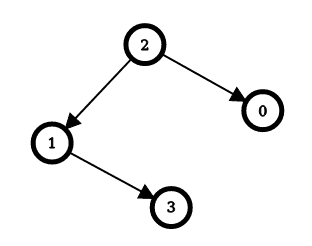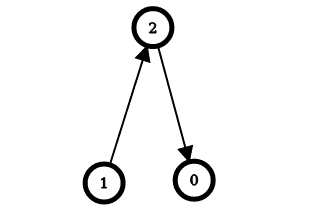# 2858. Minimum Edge Reversals So Every Node Is Reachable

## Description

There is a simple directed graph with n nodes labeled from 0 to n - 1. The graph would form a tree if its edges were bi-directional.

You are given an integer n and a 2D integer array edges, where edges[i] = [ui, vi] represents a directed edge going from node ui to node vi.

An edge reversal changes the direction of an edge, i.e., a directed edge going from node ui to node vi becomes a directed edge going from node vi to node ui.

For every node i in the range [0, n - 1], your task is to independently calculate the minimum number of edge reversals required so it is possible to reach any other node starting from node i through a sequence of directed edges.

Return an integer array answer, where answer[i] is the minimum number of edge reversals required so it is possible to reach any other node starting from node i through a sequence of directed edges.

Example 1:Input: n = 4, edges = [[2,0],[2,1],[1,3]]
Output: [1,1,0,2]
Explanation: The image above shows the graph formed by the edges.
For node 0: after reversing the edge [2,0], it is possible to reach any other node starting from node 0.
For node 1: after reversing the edge [2,1], it is possible to reach any other node starting from node 1.
For node 2: it is already possible to reach any other node starting from node 2.
For node 3: after reversing the edges [1,3] and [2,1], it is possible to reach any other node starting from node 3.


Example 2:Input: n = 3, edges = [[1,2],[2,0]]
Output: [2,0,1]
Explanation: The image above shows the graph formed by the edges.
For node 0: after reversing the edges [2,0] and [1,2], it is possible to reach any other node starting from node 0.
For node 1: it is already possible to reach any other node starting from node 1.
For node 2: after reversing the edge [1, 2], it is possible to reach any other node starting from node 2.


Constraints:

• 2 <= n <= 105
• edges.length == n - 1
• edges[i].length == 2
• 0 <= ui == edges[i] < n
• 0 <= vi == edges[i] < n
• ui != vi
• The input is generated such that if the edges were bi-directional, the graph would be a tree.

## Solutions

• class Solution {
private List<int[]>[] g;
private int[] ans;

public int[] minEdgeReversals(int n, int[][] edges) {
ans = new int[n];
g = new List[n];
Arrays.setAll(g, i -> new ArrayList<>());
for (var e : edges) {
int x = e, y = e;
}
dfs(0, -1);
dfs2(0, -1);
return ans;
}

private void dfs(int i, int fa) {
for (var ne : g[i]) {
int j = ne, k = ne;
if (j != fa) {
ans += k < 0 ? 1 : 0;
dfs(j, i);
}
}
}

private void dfs2(int i, int fa) {
for (var ne : g[i]) {
int j = ne, k = ne;
if (j != fa) {
ans[j] = ans[i] + k;
dfs2(j, i);
}
}
}
}

• class Solution {
public:
vector<int> minEdgeReversals(int n, vector<vector<int>>& edges) {
vector<pair<int, int>> g[n];
vector<int> ans(n);
for (auto& e : edges) {
int x = e, y = e;
g[x].emplace_back(y, 1);
g[y].emplace_back(x, -1);
}
function<void(int, int)> dfs = [&](int i, int fa) {
for (auto& [j, k] : g[i]) {
if (j != fa) {
ans += k < 0;
dfs(j, i);
}
}
};
function<void(int, int)> dfs2 = [&](int i, int fa) {
for (auto& [j, k] : g[i]) {
if (j != fa) {
ans[j] = ans[i] + k;
dfs2(j, i);
}
}
};
dfs(0, -1);
dfs2(0, -1);
return ans;
}
};

• class Solution:
def minEdgeReversals(self, n: int, edges: List[List[int]]) -> List[int]:
ans =  * n
g = [[] for _ in range(n)]
for x, y in edges:
g[x].append((y, 1))
g[y].append((x, -1))

def dfs(i: int, fa: int):
for j, k in g[i]:
if j != fa:
ans += int(k < 0)
dfs(j, i)

dfs(0, -1)

def dfs2(i: int, fa: int):
for j, k in g[i]:
if j != fa:
ans[j] = ans[i] + k
dfs2(j, i)

dfs2(0, -1)
return ans


• func minEdgeReversals(n int, edges [][]int) []int {
g := make([][]int, n)
for _, e := range edges {
x, y := e, e
g[x] = append(g[x], int{y, 1})
g[y] = append(g[y], int{x, -1})
}
ans := make([]int, n)
var dfs func(int, int)
var dfs2 func(int, int)
dfs = func(i, fa int) {
for _, ne := range g[i] {
j, k := ne, ne
if j != fa {
if k < 0 {
ans++
}
dfs(j, i)
}
}
}
dfs2 = func(i, fa int) {
for _, ne := range g[i] {
j, k := ne, ne
if j != fa {
ans[j] = ans[i] + k
dfs2(j, i)
}
}
}
dfs(0, -1)
dfs2(0, -1)
return ans
}

• function minEdgeReversals(n: number, edges: number[][]): number[] {
const g: number[][][] = Array.from({ length: n }, () => []);
for (const [x, y] of edges) {
g[x].push([y, 1]);
g[y].push([x, -1]);
}
const ans: number[] = Array(n).fill(0);
const dfs = (i: number, fa: number) => {
for (const [j, k] of g[i]) {
if (j !== fa) {
ans += k < 0 ? 1 : 0;
dfs(j, i);
}
}
};
const dfs2 = (i: number, fa: number) => {
for (const [j, k] of g[i]) {
if (j !== fa) {
ans[j] = ans[i] + k;
dfs2(j, i);
}
}
};
dfs(0, -1);
dfs2(0, -1);
return ans;
}